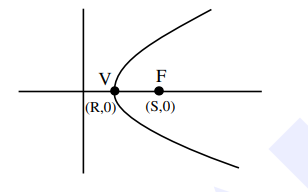# The length of the latus rectum of a parabola,`
Question:

The length of the latus rectum of a parabola, whose vertex and focus are on the positive $x$-axis at a distance $R$ and $S(>R)$ respectively from the origin, is :

1. $4(\mathrm{~S}+\mathrm{R})$

2. $2(\mathrm{~S}-\mathrm{R})$

3. $4(\mathrm{~S}-\mathrm{R})$

4. $2(\mathrm{~S}+\mathrm{R})$

Correct Option: , 3

Solution:$\mathrm{V} \rightarrow$ Vertex

$\mathrm{F} \rightarrow$ focus

$\mathrm{VF}=\mathrm{S}-\mathrm{R}$

So latus rectum $=4(\mathrm{~S}-\mathrm{R})$